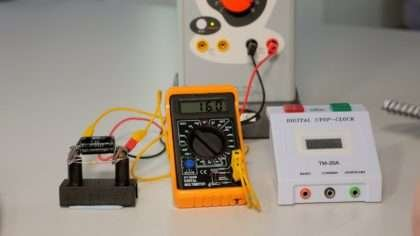Share with friends

Physics is one of the most important subjects in Class 12. As the CBSE exam approaches, students get busy preparing for different subjects. But an essential part of the CBSE exam is the practical exams which consist of 30 marks.

Students must know all the experiments along with theorems, laws, and numerical to understand all the concepts of 12th standard physics in a detailed way. Two experiments (8 + 8 marks) are asked from each section in the practical exam. The experiment records and activities consist of 6 marks, the project has 3 marks and viva on the experiment consist of 5 marks.

The Physics Practicals For Class 12 CBSE is given here so that students can understand the experiments in a better way. Students are suggested to study the theory and law behind the experiment properly before performing the experiment.

Practical Examination for Visually Impaired Students of Classes XI and XII Evaluation Scheme

Time Allowed: Two hours

Identification/Familiarity with the apparatus Written test (based on given/prescribed practicals) Practical Record

Max. Marks: 30

5 marks

10 marks

5 marks

10 marks

30 marks

General Guidelines

1. The practical examination will be of two hour duration.
2.  A separate list of ten experiments is included here.
3. The written examination in practicals for these students will be conducted at the time of practical examination of all other students.
4. The written test will be of 30 minutes duration.
5. The question paper given to the students should be legibly typed. It should contain a total of 15 practical skill based very short answer type questions. A student would be required to answer any 10 questions.
6. A writer may be allowed to such students as per CBSE examination rules.
7. All questions included in the question papers should be related to the listed practicals. Every question should require about two minutes to be answered.
8. These students are also required to maintain a practical file. A student is expected to record at least five of the listed experiments as per the specific instructions for each subject. These practicals should be duly checked and signed by the internal examiner. The format of writing any experiment in the practical file should include aim, apparatus required, simple theory, procedure, related practical skills, precautions etc.
9. 9. Questions may be generated jointly by the external/internal examiners and used for assessment.
10. The viva questions may include questions based on basic theory/principle/concept, apparatus/ materials/chemicals required, procedure, precautions, sources of error etc.

Class XII

1. Items for Identification/ familiarity with the apparatus for assessment in practicals (All experiments)

Meter scale, general shape of the voltmeter/ammeter, battery/power supply, connecting wires, standard resistances, connecting wires, voltmeter/ammeter, meter bridge, screw gauge, jockey Galvanometer, Resistance Box, standard Resistance, connecting wires, Potentiometer, jockey, Galvanometer, Lechlanche cell, Daniell cell [simple distinction between the two vis-a-vis their outer (glass and copper) containers], rheostat connecting wires, Galvanometer, resistance box, Plug-in and tapping keys, connecting wires battery/power supply, Diode, Resistor (Wire-wound or carbon ones with two wires connected to two ends), capacitors (one or two types), Inductors, Simple electric/electronic bell, battery/power supply, Plug-in and tapping keys, Convex lens, concave lens, convex mirror, concave mirror, Core/hollow wooden cylinder, insulated wire, ferromagnetic rod, Transformer core, insulated wire.

• List of Practicals
1. To determine the resistance per cm of a given wire by plotting a graph between voltage and current.
1. To verify the laws of combination (series/parallel combination) of resistances by

Ohm’s law.

• To find the resistance of a given wire using a meter bridge and hence determine

the specific resistance (resistivity) of its material.

• To compare the e.m.f of two given primary cells using a potentiometer.
• To determine the resistance of a galvanometer by half deflection method.
• To identify a resistor, capacitor, inductor and diode from a mixed collection of such items.
• To understand the principle of (i) a NOT gate (ii) an OR gate (iii)an AND gate and to make their equivalent circuits using a bell and cells/battery and keys /switches
• To observe the difference between
• a convex lens and a concave lens
• a convex mirror and a concave mirror and to estimate the likely difference between the power of two given convex /concave lenses.